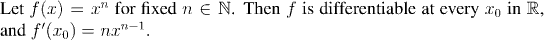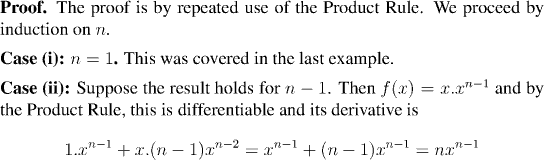Analysis WebNotes
arrow_back arrow_forward

# Class Contents

## The Derivative and its Arithmetic Properties

The derivative of a function is a particular kind of limit that measures the slope of the graph of a function at a point. To calculate the slope of the function at the point x=a one computes the slope of the secant line: the straight line joining the points (a,f(a)) and (x,f(x)), and looks for the limit of this quantity as the point x gets closer and closer to x=a.
Remark:

Say that a point x in a set D is an interior point of D if there is an open interval (a,b) which contains x and which is contained in D.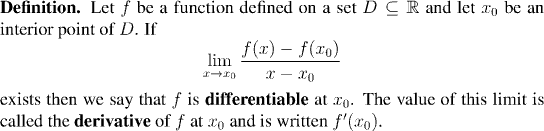Remark:

Why do we insist that the point x0 is an interior point? In order for the difference quotient to make sense, f must be defined at x0 and so x0 must belong to D. On the other hand, in order for the limit to make sense, x0 must be a limit point of D. It is possible for a point to belong to both D and D' and still not be an interior point, but since most of the results on differentiability require that f actually be differentiable on an interval, it makes sense to only consider dereivatives at interior points of D. Of course an interior point will always belong to both D and D'.

Remark:

The quotient in the definition of the derivative is called the difference quotient for f at x0. Note that it is exactly the slope of the secant line joining (x, f(x)) and (x0, f(x0)).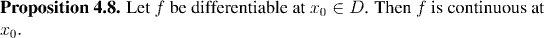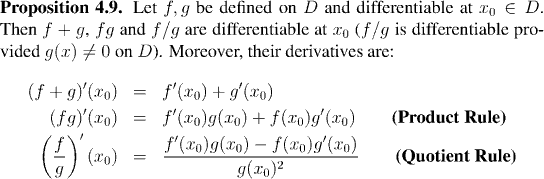Remark:

You should observe that Theorem 4.9 is the latest in a series of theorems which establish relationships between limits of functions and algebraic combinations of those functions. The previous results in this direction have been Theorem 3.9, Theorem 3.11, Theorem 4.1 (which we used in the present theorem), Theorem 4.4, and Theorem 4.5.

Example: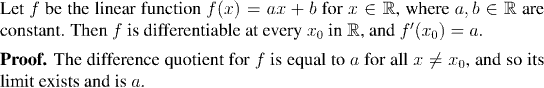Remark:

In particular, the derivative of a constant function is zero, and the derivative of f(x)=x is 1.

Example: